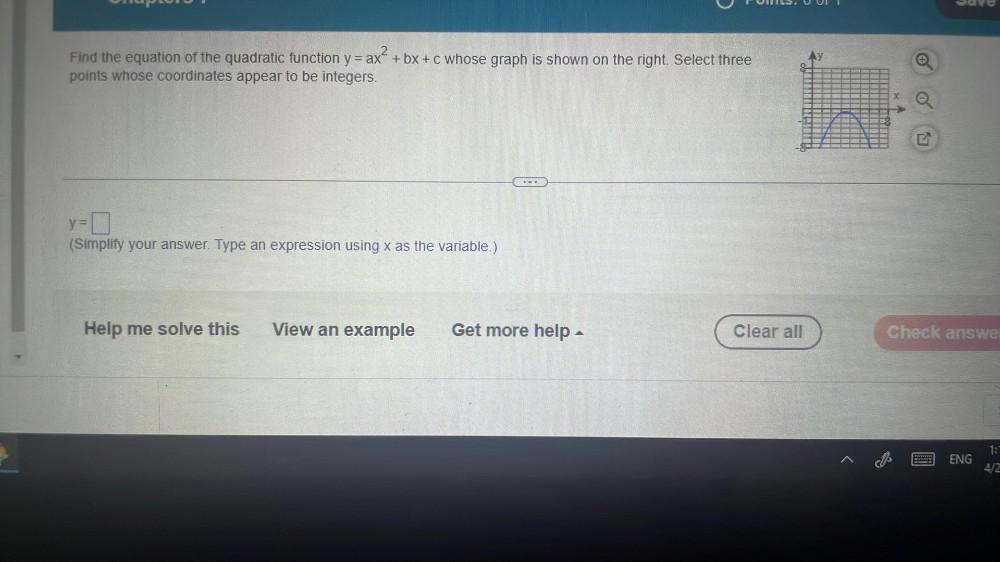Question:

# TUI. UIT e Find the equation of the quadratic function y = ax' + bx+c whose graph is shown on the right. Select three ? points wTUI. UIT e Find the equation of the quadratic function y = ax' + bx+c whose graph is shown on the right. Select three ? points whose coordinates appear to be integers. N y=0 = (Simplify your answer. Type an expression using x as the variable.) Help me solve this View an example Get more help - Clear all Check answe ENG 1: 4/2